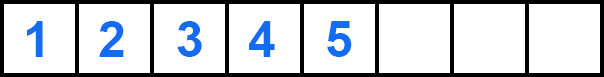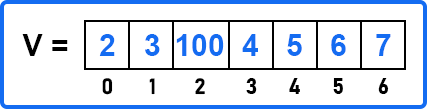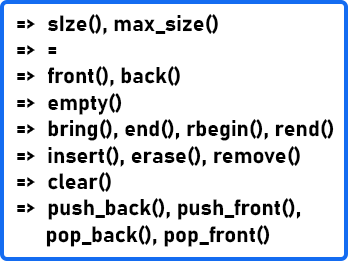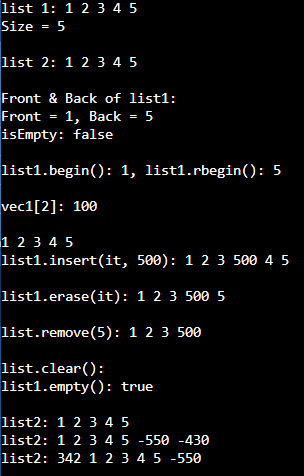# List Class in C++

## List Class in C++ with Examples:

In this article, I am going to discuss List Class Functions in C++ with Examples. Please read our previous article, where we discussed Vector and Vector Functions in C++ with Examples.

##### List Class in C++:

In the last article, we had seen std::vector. The List is also a sequence container. Lists are optimized for rapid insert and delete operations. In lists, data are stored non-contiguously and in vectors, we saw that we have a contiguous chunk of data. You can think of it as some sort of array.If you insert some element in between the elements, everything else needs to be shifted. So here insert is of the order of the number of elements i.e. O(n). You can think of it as a doubly linked list so that is why it allows iteration in both directions. And in the ‘introduction to containers’ article, we also said that there is also a forward list. So if you just need a forward iteration or a singly linked list then you should use std::forward_list.

But in the list, both iterations are possible. It does not support random access. In vectors, we can access any element using the Index number.If we have a vector ‘v’ and the index starts from 0 to 6 then we can access any element i.e., v , v . It would be done in constant time but in the list that is not the case. You need to traverse through the list. So, you have to keep a pointer to the beginning or an iterator to the beginning of the list and then increment the iterator. you can keep track of the count and then fetch the value.

If you want to find any element or check for any element in the list then you will start from the beginning. Then you will dereference the iterator and check whether it is that element or not. If not, increment the iterator and check again, and so on. And you also have to check whether you don’t reach the end of the list. So, end () is pointing to one hypothetical position which is after the last element. That is used for bound checking that we have reached the end of the list. We will see an example of all of this in detail. This gave you some idea about the list. Now let’s see the main functions.

##### List Functions in C++:

Please have a look at the below image which shows the list of functions that are available in the List class.The List’s functions are very similar to the vector’s functions. In fact, many of these functions are common to most of the sequence containers.

Clear() – it can be a very useful function. This function will clear the complete container. Suppose we have a vector v with some values,If we write v.clear(), v will become an empty vector. So, this is very handy and it is used quite a lot.

Size() – it is present in vector also. It will give you the number of elements that you have inserted.

max_size() – it is present in vectors. It will return the maximum number of elements that can be held by the vector container or list container. It is not very useful for us.

= (equal) – with an equal you can assign elements using an initializer list. Let us say we have a list,

list<int> l1 = {1, 3, 5, 7};

We can create another list of the same type let us call it l2,

list<int> l2 = l1;

We have assigned l2 to l1. So, you are allowed to assign an old list to a new list and it will create a copy and not the reference

front(), back() These are very similar to vector’s functions. The Front function will return the first element and the back function will return the last element.

empty() – It is used to check whether the list is empty or not and we use this function a lot in our code. This will return Boolean True or false when the list is empty or not.

begin(), end() The begin() function returns an iterator to the first element, and the end() function returns the iterator to the one position past the last element. It is used for bound checks. we generally do not dereference it, unlike the begin iterator which is actually pointing to one of the elements so we can dereference it.

rbegin(), rend() – The rbegin means we want to traverse in the reverse direction. so when we want to traverse in the reverse direction, whatever is the last element, r begin will point there. We will give examples of this in running code and then you will see it in action. Similarly, rend points one position before the first element.

Insert(), erase(), remove() – suppose we want to insert at the third position so you need an iterator to the third element then we will write insert (it, 100). 100 will be inserted at the 3rd position. So one element has been inserted at the third position. Now, what is the difference between erase and remove? Erase function is just like the insert. Here you have to pass the iterator in erase function. Suppose we want to erase the third element so we will write, n.erase (it). You can also pass a range i.e. n.erase (it1, it2).

Now suppose we want to remove value 4 in vector v. So we will write v.remove (4). It will find 4 and remove that from the vector. So what will it internally do? It will start from the beginning and compare every element with four and when it will reach 4, it will remove that element. So this is expected to take linear time and all of these expected times you can guess since you are allowed to only go one at a time. So once you are given the iterator to that element that node or pointer to that node, for example, you are straight away given one iterator pointing to four. Now you want to remove it. So here it will call erase function so it will remove it in constant time but if you are not given that you will need to traverse everything or let’s say you call remove then it has to first find and then remove.

clear() – clear can be very handy to clear the complete container or remove everything. So it will make the container empty.

push_back() – it will push an element in the end. You have to pass a value here let’s say v.push_back (40) so it will add 40 in the end.

push_front() – it will add the element at the beginning i.e. v.push_front (10).

pop_back() – it will remove the element from the end.

pop_front() – it will remove the element from the beginning.

So, these are some specialized functions. These are the most frequently used so we have specialized values for these specialized names for this.

##### Example to Understand List Class Functions in C++:
```#include <iostream>
#include <list>
#include <vector>

using namespace std;

void print_list(list<int>& ll){
for(list<int>::iterator it = ll.begin(); it != ll.end(); ++it)
cout << *it << " ";
cout << endl;
}

int main()
{
// create list 1
list<int> list1 = {1, 2, 3, 4, 5};
cout << "list 1: ";
print_list(list1);
cout << "Size = " << list1.size() << endl;
cout << endl;

// create list 2 by coping the elements of list 1
list <int> list2 = list1;
cout << "list 2: ";
print_list(list2);
cout << endl;

cout << "Front & Back of list1: " << endl;
cout << "Front = " << list1.front() << ", Back = " << list1.back() << endl;
// To check whether the list1 is empty or not
cout << "isEmpty: " << std:: boolalpha << list1.empty() << endl;
cout << endl;

cout << "list1.begin(): " << *list1.begin() << ", list1.rbegin(): " << *list1.rbegin() << endl;
cout << endl;

// Direct accessing vector elements but in list we cannot direct access to any element
cout << "vec1: ";
vector<int> vec1 = {1,2,3,4,5};
vec1.insert(vec1.begin() + 2, 100);
cout << vec1 << endl;
cout << endl;

// insert the given number at given position in list
print_list(list1);
cout << "list1.insert(it, 500): ";
list<int>::iterator it = list1.begin();
while(*it != 4 && it != list1.end())
it++;
list1.insert(it, 500);
print_list(list1);
cout << endl;

// erase the element where iterator is pointing
cout << "list1.erase(it): ";
list1.erase(it);
print_list(list1);
cout << endl;

// remove the given element from the list
cout << "list.remove(5): ";
list1.remove(5);
print_list(list1);
cout << endl;

// clear the list
cout << "list.clear(): ";
list1.clear();
print_list(list1);
cout << "list1.empty(): " << std::boolalpha << list1.empty() << endl;
cout << endl;

cout << "list2: ";
print_list(list2);
list2.push_back(-550);
list2.push_back(-430);

cout << "list2: ";
print_list(list2);
list2.pop_back();
list2.push_front(342);

cout << "list2: ";
print_list(list2);

return 0;
}
```
###### Output: